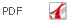J. Iran. Chem. Soc., Vol. 7, No. 4 December 2010, pp.986~994.

Current location: JICS Archive > Vol. 7 > No. 4 > Articles : 25

# The Combinatorial Factor Method for Investigation of Order-Disorder in Two-Dimensional Binary Alloy

## S. Ranjbara,* and S.J. Hossinib

### aChemistry College, Razi University, Kermanshah, Iran bChemistry Department Azad University, Gachsaran, Iran

The aim of this study was to investigate order-disorder in the two-dimensional AB alloy to find out whether the number of components, NA and NB, was equal or not. To this end, using the nearest neighbor interactions, first, we applied a two-dimensional lattice which consisted of infinite rows, R and columns, L, so that, RL = NA + NB. For such a model, using the combinatorial factor method, we derived an exact equation for the partition function. Because, the derived partition function was very complicated, the thermodynamic properties of the lattice could not be calculated; however, but these properties could be estimated for the models with a limited number of rows. Our results show that, for models with finite number of rows, for each mole fraction of A in the specific temperature, a phase transition, like Onsager transition, takes place.

Keywords: Isin model, Spin glasses, Binary alloy, Combinatorial factor, Phase transition